# GSEB Solutions Class 11 Maths Chapter 7 Permutations and Combinations Miscellaneous Exercise

Gujarat Board GSEB Textbook Solutions Class 11 Maths Chapter 7 Permutations and Combinations Miscellaneous Exercise Textbook Questions and Answers.

## Gujarat Board Textbook Solutions Class 11 Maths Chapter 7 Permutations and Combinations Miscellaneous Exercise

Question 1.
How many words, with or without meaning, each of 2 vowels and 3 consonents can be formed from the letters of the word DAUGHTER?
Solution:
The word DAUGHTER consists of 3 vowels and 5 consonants. 2 vowels and 3 consonants may be selected in 3C2 × 5C2 ways.
= 3C1 × 5C2 ways = $$\frac{3}{1}$$ × $$\frac{5×4}{1×2}$$ = 30 ways.
5 letters can be arranged in 5! ways.
or in 5 × 4 × 3 × 2 × 1 = 120 ways.
∴ Number of words formed by 2 vowels and 3 consonants which are selected from the word DAUGHTER = 30 × 120 = 3600.Question 2.
How many words with or without meaning can be formed with letters of the word EQUATION at a time so that vowels and consonants occur together?
Solution:
The word EQUATION consists of 5 vowels and 3 consonants. 5 words can be arranged in 5! = 120 ways.
3 consonants can be arranged in 3! = 6 ways.
The two block of vowels and consonants can be arranged in 2! = 2 ways.
∴ The number of words which can be formed with letters of the word EQUATION so that vowels and consonants occur together
= 120 × 6 × 2
= 1440.Question 3.
A committee of 7 has to be formed from 9 boys and 4 girls. In how many ways, can this be done, when the committee consists of
(i) Exactly 3 girls?
(ii) At least 3 girls?
(iii) At most 3 girls?
Solution:
(i) A committee consisting of 3 girls and 4 boys can be formed in 4C3 × 9C4 = $$\frac{4}{1}$$ × $$\frac{9×8×7×6}{1.2.3.4}$$ ways = 504 ways.

(ii) A committee having at least 3 girls will consists of
(a) 3 girls 4 boys
(b) 4 girls 3 boys.
This can be done in 4C3 × 9C4 + 4C4 × 9C3 ways
= $$\frac{4}{1}$$ × $$\frac{9×8×7×6}{1×2×3×4}$$ + 1 × $$\frac{9×8×7}{1×2×3}$$ ways
= 504 + 84 ways
= 588 ways.

(iii) A committee having at most 3 girls will consist of
(a) No girl, 7 boys
(b) 1 girl, 6 boys
(c) 2 girls, 5 boys
(d) 3 girls, 4 boys
This can be done in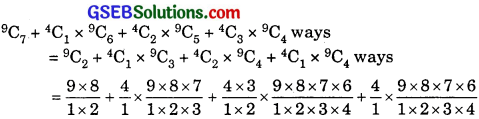= 36 + 4 × 84 + 6 × 126 + 4 × 126 ways
= 36 + 336 + 126(6 + 4) ways
= 36 + 336 + 1260 ways
= 1632.Question 4.
If the different permutations of all the letters of the word EXAMINATION are listed as in Dictionary, how many words are there in this list before the first word starting with E?
Solution:
Words starting with A are formed with the letters 2 I’s, 2N’s, A, E, X, M, T, O
Number of words formed by these letters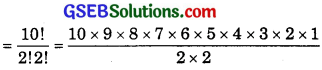= 10 × 9 × 8 × 7 × 6 × 5 × 3 × 2 × 1
= 907200.
Then, the words starting with E, I, M, N, O, T and X will be formed.
∴ Number of words before the first word starting with E is formed = 907200.

Question 5.
How many 6 digit numbers can be formed from the digits 0, 1, 3, 5, 7 and 9 which are divisible by 10 and no digit is repeated?
Solution:
A number is divisible by 10, if zero occurs at the unit’s place. Now, we have to fill up 5 places with the digits 1, 3, 5, 7 and 9.
This can be done in 5! = 120 ways.
∴ Required number of numbers = 120.Question 6.
The English alphabet has 5 vowels and 21 consonants. How many words with two different vowels and two different consonants can be formed from the alphabet?
Solution:
2 vowels can be chosen in 5C2 ways.
2 consonants can be chosen in 21C2 ways.
4 letters can be arranged in 4! ways.
∴ The number of words consisting of 2 vowels and 2 consonants
= 5C2 × 21C2 × 4!.
= $$\frac{5×4}{1×2}$$ × $$\frac{21×20}{1×2}$$ × 24
= 10 × 210 × 24
= 50400.

Question 7.
In an examination, a question paper consists of 12 questions, divided into two parts, i.e., Part I and Part II, containing 5 and 7 questions respectively. A student is required to attempt 8 questions in all, selecting at least 3 from each part. In how many ways, can a student select the questions?
Solution:
Student may select 8 questions according to following scheme: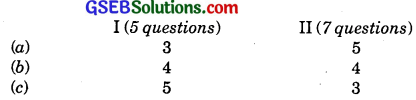If p is the required number of ways, then
p = C(5, 3) × C(7, 5) + C(5, 4) × C(7, 4) + C(5, 5) × C(7, 3).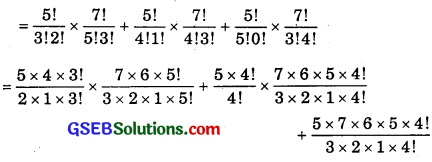= 10 × 7 + 5 × 35 + 5 × 35
= 70 + 175 + 175
= 420 ways.Question 8.
Determine the number of 5-cards combinations, out of a deck of 52 cards, if each selection of 5 cards has exactly one king?
Solution:
1 king can be selected in 4C1 ways.
4 other cards can be selected in 48C4 ways.
∴ No. of card combinations, out of a deck of 52 cards, each combination having exactly one king
= 4C1 × 48C4.

Question 9.
It is required to seat 5 men and 4 women in a row so that the women occupy the even places. How many such arrangements are possible?
Solution:
According to the problem, women will sit at the even places (2, 4, 6 and 8). They can be seated in P(4, 4) = 4! = 24 ways.
Men will sit on the odd seats (i.e., 1, 3, 5, 7 and 9).
They can sit on these seats in P(5, 5) = 5! = 120 ways.
If total number of ways p, then p = 24 × 120 = 2880.Question 10.
From a class of 25 students, 10 are to be chosen for an excursion party. There are 3 students who decide that either all of them will join or none of them will join. In how many ways can the excursion party be chosen?
Solution:
There are two cases.
(a) If the 3 students join the excursion party, then the number of combinations will be p1 = C(22, 7).
(b) If the 3 students do not join the excursion party.
Then, the number of combinations p2 = C(22, 10).
If p is the combination of choosing the excursion party, then
p = p1 + p2 = C(22, 7) + C(22,10).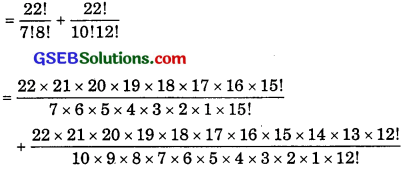= 817190.Question 11.
In how many ways, can the letters of the word ASSASSINATION be arranged so that all the S’s are together?
Solution:
The word ASSASSINATION has 4S, 3A, 21,2N, T, O. Out of these 4S are to be together. This is considered as one block as 1 letter.
Now, we have : 3A, 21, 2N, (4S), T, O
∴ Number of words
= Number of permutations of 3A, 21, 2N (4S), T, O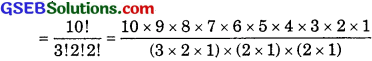= 10 × 9 × 8 × 7 × 6 × 5 × 4 × 3 × 2 × 1
= 151200.